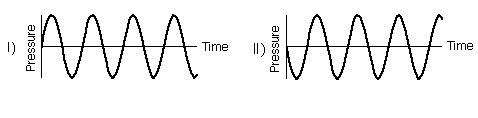TUTORIAL QUESTIONS 190-200

Questions:
190: Sounds of two complex waves
193: What are the relative phases?
195: Frequency in a complex wave
200: Period of the harmonics

Question 190.  If we play the sounds made by two (different) complex waves made up of 200 and 400 Hz sinusoidal waves, they would sound different.

a) true
b) false

Fundamentals of Sound reference:Sec. 6-C.

Question 193.  The two sinusoidal waves shown below are in phase.

a) true
b) falseFundamentals of Sound reference:Sec. 6-A.

Question 195.  One way to find the frequencies that make up a complex, repeating wave, is to find its repeat time, and take its reciprocal.

a) true
b) false

Fundamentals of Sound reference:Secs. 6-C, 7-B.

Question 200.  For standing sinusoidal waves on a string tied at both ends, the period of the first harmonic is always twice as long as the period of the second harmonic.

a) true
b) false

Fundamentals of Sound reference:Secs. 2-C, 6-D.